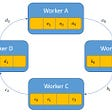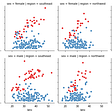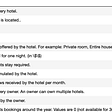# 1. Accuracy:

Accuracy is defined as the number of correctly classified points divided by the total number of points in the test set. Accuracy lies between 0 to 1 where the accuracy of 0 is considered to be bad and the accuracy of 1 is considered to be better. Understanding: Let's say your test set has 100 points of which you had 60 positive points and 40 negative points. Out of 60 positive points the model predicted 53 positive points and did an error on 7 points and of 40 negative points, the model predicted 35 points to be negative and 5 points to be positive. Now, we make total errors of 12points and correctly classified points are 88. Which means the accuracy is 88%.

Problems with Accuracy (Imbalanced data): In test data, assume 90% of data to be negative class and 10%of data belongs to the positive class. This is called imbalanced data. Having a dumb model ‘M’, which says given any query point ‘xq’ return it as negative. Here if we run model M on imbalanced data, the accuracy will be 90%. We get a high accuracy for a dumb model which means you should never use accuracy measure when you have an imbalanced dataset.

# 2. Confusion Matrix:

A confusion matrix is a N X N matrix or a table that is often used to describe the performance of a classification model. It also allows the visualization of the performance of an algorithm. It gives us insight into the type of errors made by the classifier.

Consider a binary classification task having a 2 X 2 matrix.

Here we have actual class value and predicted class value. In each of the classes, we have a Positive class as ‘1' and a Negative class as ‘0'.

Definition of the Terms:

1. True Positive(TP): For the actual class to be positive, the predicted class is also positive. For eg; If the alarm goes on in case of fire, here the system predicted fire to be positive which is true.
2. False Positive(FP): For the actual class to be negative, the predicted class is positive. For eg; If the alarm goes on and there is no fire, here the system predicted fire to be positive which is false.
3. False Negative(FN): For the actual class to be positive, the predicted class is negative. For eg; If the alarm does not go on but there was a fire, here the system predicted fire to be negative which is false.
4. True Negative(TN): For the actual class to be negative, the predicted class is also negative. For eg; If the alarm does not go on and there was no fire, here the system predicted fire to be negative which is true.
5. True Positive Rate(TPR): Calculated as the total number of correct positive predictions divided by the total number of positive. (TPR = TP/FN+TP).
6. True Negative Rate(TNR): Calculated as the total number of correct negative predictions divided by the total number of negative. (TNR = TN/TN+FP).
7. False Positive Rate(FPR): Calculated as the total number of correct positive predictions divided by the total number of positive. (FPR = FP/TN+FP).
8. False Negative Rate(FNR): Calculated as the total number of correct positive predictions divided by the total number of positive. (FNR = FN/FN+TP).

Note: In the Confusion matrix, the diagonal elements of the matrix also known as the principal diagonal elements must be high, and the rest (ie; off-diagonal elements) should be small if the model is good.

Example: In the medical domain to diagnose a person has cancer or not. Here from the above matrix:

a) When a person has cancer and the Model predicts cancer then the person gets the treatment(TPR must be very high).

b) When a person has cancer and the Model predicts a person doesn’t have cancer, then the person could die because of a false test(FNR should be low or zero).

c) When a person doesn’t have cancer, but the model says it has then it's ok that the person will go through more tests(FPR is high).

d) When a person doesn’t have cancer and the model also says it doesn’t have cancer, then we can say the model is good(TNR is high).

# 3. Precision, Recall, and F1 Score:

Precision: It is defined as the ratio of the total number of correctly classified positive points to the total number of predicted positive points. Precision says that of all the points the model Predicted to be positive, what percentage of them are truly positive. High precision indicates a small number of False Positive points whereas low precision indicates a large number of False Positive points.

Precision = TP/(FP+TP)

Recall: It is defined as the ratio of the total number of correctly classified positive points to the total number of actual positive points. The Recall says that of all the positive points, how many of them are predicted to be positive.

Recall = TP/(FN+TP)

F1-Score: The F1 score is also known as F-Measure which is the harmonic mean of both the metrics precision and recall. F1-Score is high when precision is high and recall is high.

# 4. Log-Loss:

Log Loss is the most popular performance metric used in classification problems. Log Loss is defined as the negative average of the log of corrected predicted probabilities for each instance. Or we can say Log loss is the average of the negative log of the probability of correct class label.

Intuition: Log-Loss uses the exact probabilities scores. For binary classification, Inputs(x) = x1, x2, x3, x4 and Class Label(y) = 1, 1, 0, 0 and their respective probabilities are 0.9, 0.6, 0.1, 0.4 The formula of log-loss:-

Where,

In the actual class, let yi = 1 for positive class and yi = 0 for the negative class

p(yi) is the predicted probability of positive class

1-p(yi) is the predicted probability of negative class

Now calculating log-loss using the formula:

Log loss(1, 0.9) = 0.0457

Log loss(1, 0.6) = 0.22

Log loss(0, 0.1) = 0.0457

Log loss(0, 0.4) = 0.22

Here, log loss lies between 0 to infinity, and also smaller the log loss the better is the performance of the model.

In class label 1, 0.9 is close to 1 and the error is less (0.0457) but 0.6 is far from 1 and the error is more(0.22).

In class label 0, 0.1 is close to 0 and the error is less(0.0457) but 0.4 is far from 0 and error is more(0.22)

Note: When you have probability scores, log loss is the most powerful metric for both binary and multiclass classification.

# 5. Area Under ROC Curve(AUC):

The area under the curve is a widely used performance metric for only binary classification. ROC is a probability curve that classifies the randomly chosen positive class higher than the negative class. AUC curve is the total area under the whole curve and it has a range of 0 to 1. The greater the value, the better is the performance of the model.

# 6. Mean Absolute Error:

Mean absolute error is used in regression problems. It is the average of the difference between the original values and predicted values. It gives the deviation of how far the predicted output is from the actual output. Mathematically,

# 7. Root Mean Squared Error:

RMSE is the most popular performance metric used in regression problems. The mean square error takes the average of the square of the difference between the original values and the predicted values. As RMSE is highly affected by outlier values, make sure you have removed all the outliers from your data. The formula for RMSE metric:

Where n is the total number of data points, yi is the actual output values, and yi^ is the predicted output values.

These are some of the commonly used performance metrics for evaluating your machine learning models. Hope you will find this article useful.

Happy Learning!

--

--

--

## More from Machine Learning Community

The goal is to learn and share the concept, ideas, and code of Machine Learning, Deep Learning, Natural Language Processing, Computer Vision, Statistics, and Python.

## Must-read Guide to Hypothesis Tests You Will Never Use## How to tune a Random Forest model## Practical ML Part 1: COVID-19 in Brazil## Visual intuition on ring-Allreduce for distributed Deep Learning## Machine learning## Quick Introduction to TensorFlow ( For Beginners )## Image Recognition using Core ML and Vision Framework## Personalizing Multiple Page Sections on Discovery+ via Sequence Aware Multi-Task Learning## Sabita Rajbanshi

Writing Towards Machine Learning and Artificial Intelligence.

## Naïve Bayes : A Probabilistic Approach — Analytics Mag## Predicting Insurance Cost: A machine learning and an exploratory approach.## Predicting Hotel Yearly Availability : ML Challenge## Data Transformation in Machine Learning Part-II